• 三维极坐标系
千次阅读
2021-04-18 15:43:55

3D Polar Plot

The polar axis can be placed at the minimum, maximum or mean value of Zp at the largest radius, at the top or bottom of the plot box, at a user specified location, or it can follow the surface at the perimeter of the data. The polar axis tick mark spacing can be adjusted with the 'TickSpacing' property.

The default polar axis orientation is that zero degrees is along the +X axis and increasing angles are counter-clockwise. The 'PolarDirection' property can be used to change this to a compass style plot with zero degrees along the +Y axis and increasing angles going clockwise around the pole.

Default surface coloring is according to the values in Zp. This can be changed by supplying a matrix the same size as Zp as the value of the 'ColorData' property.

A scaling parameter can be specified to interpolate the data onto a finer or coarser mesh. The output Cartesian data is returned in three matrices.

更多相关内容
• MATLAB三维绘图不论是surf还是mesh都是在笛卡尔坐标中进行，没有专门的三维极坐标绘图。以天线三维方向图为例，先将极坐标转换为笛卡尔坐标，然后使用surf在MATLAB中绘制，与HFSS图形对比，得到一致结果
• 文章目录写在前面：一、三维立体图像1、三维线框2、三维曲面3、三维散点二、极坐标系三、半对数坐标 写在前面： ...获得三维坐标轴：ax = mp.gca(projection=‘3d’) 1、三维线框 格式：ax.plot_wireframe

## 写在前面：

import numpy as np
import matplotlib.pylab as mp
因此文章中的np就代表numpy库，mp就代表pylab绘图库

### 一、三维立体图像

• 导入类：from mpl_toolkits.mplot3d import axes3d
• 获得三维坐标轴：ax = mp.gca(projection=‘3d’)

#### 1、三维线框

• 格式：ax.plot_wireframe(x, y, z, rstride=行距, cstrid=列距, linewidth=线宽, color=颜色)
• 测试代码：
import numpy as np
import matplotlib.pylab as mp
from mpl_toolkits.mplot3d import axes3d

n = 1000
# 栅格化：两组1000个-3到3的一维数组成x和y，会形成1000*1000个焦点的二维数组
x, y = np.meshgrid(np.linspace(-3, 3, n),
np.linspace(-3, 3, n))

# uniform：均匀分布，每个随机数概率一样在0.5和1.0之内产生n个随机数
y1 = (1 - x/n) * np.random.uniform(0.5, 1.0, n)  # (1 - x/n)为了方便显示
y2 = (1 - x/n) * np.random.uniform(0.5, 1.0, n)
z = (1 - x/2 + x**5 + y**3) * \
np.exp(-x**2 - y**2)
# 换行做运算需要用\隔开，exp是底数为x指数是-x**2 - y**2

# 图像属性
mp.figure("3D Wireframe")

# 创建3D坐标轴
ax = mp.gca(projection='3d')

mp.title("3D Wireframe", fontsize=20)  # 图像标题

# X、Y、Z坐标轴文本
ax.set_xlabel('X', fontsize=14)
ax.set_ylabel('Y', fontsize=14)
ax.set_zlabel('Z', fontsize=14)

mp.tick_params(labelsize=10)  # 刻度标签大小

# 绘制三维线框图像
ax.plot_wireframe(x, y, z, rstride=30, cstride=30, linewidth=0.5, color='orangered')

mp.show()

• 测试效果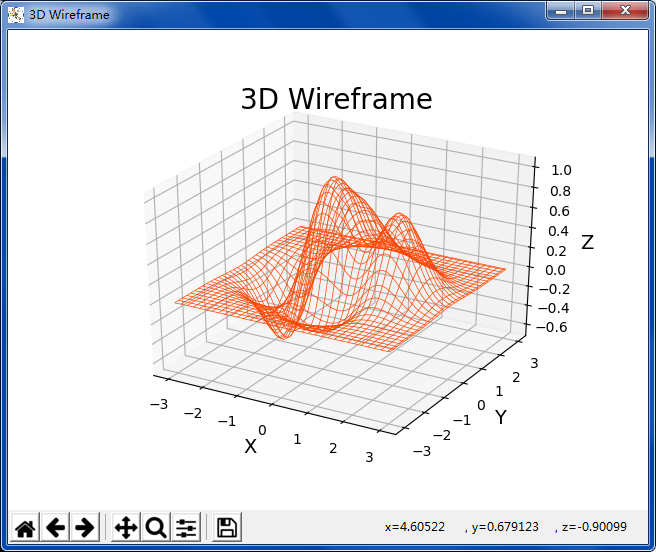#### 2、三维曲面

• 格式：ax.plot_surface(x, y, z, rstride=行距, cstrid=列距, cmap=颜色映射)
• 测试代码：
import numpy as np
import matplotlib.pylab as mp
from mpl_toolkits.mplot3d import axes3d

n = 1000
# 栅格化：两组1000个-3到3的一维数组成x和y，会形成1000*1000个焦点的二维数组
x, y = np.meshgrid(np.linspace(-3, 3, n),
np.linspace(-3, 3, n))

# uniform：均匀分布，在0.5和1.0之内产生n个随机数
y1 = (1 - x/n) * np.random.uniform(0.5, 1.0, n)  # (1 - x/n)为了方便显示
y2 = (1 - x/n) * np.random.uniform(0.5, 1.0, n)
z = (1 - x/2 + x**5 + y**3) * \
np.exp(-x**2 - y**2)
# 换行做运算需要用\隔开，exp是底数为x指数是-x**2 - y**2

# 图像属性
mp.figure("3D Surface")

# 创建3D坐标轴
ax = mp.gca(projection='3d')

mp.title("3D Surface", fontsize=20)  # 图像标题

# X、Y、Z坐标轴文本
ax.set_xlabel('X', fontsize=14)
ax.set_ylabel('Y', fontsize=14)
ax.set_zlabel('Z', fontsize=14)

mp.tick_params(labelsize=10)  # 刻度标签大小

# 绘制三维线框图像：行距列距越小，画的图越密集
ax.plot_surface(x, y, z, rstride=10, cstride=10, cmap='jet')

mp.show()

• 测试效果：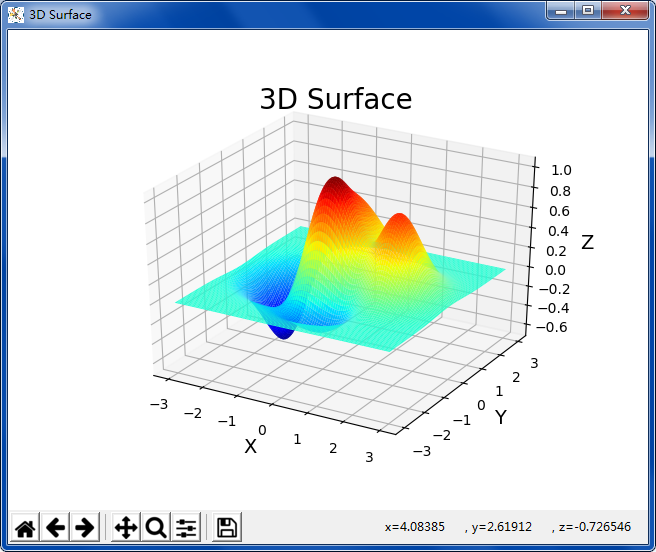#### 3、三维散点

• 格式：ax.scatter(x, y, z, s=大小, c=颜色, marker=点型)
• 测试代码：
import numpy as np
import matplotlib.pylab as mp
from mpl_toolkits.mplot3d import axes3d

# 获得1000个使用随机作为服从正态分布的数据样本（数组）
n = 1000
x = np.random.normal(0, 1, n)  # 一参为标准差，二参为分布平坦性（越大越分散，越小越集中），三参为点的个数
y = np.random.normal(0, 1, n)
z = np.random.normal(0, 1, n)
d = np.sqrt(x ** 2 + y ** 2 + z ** 2)  # 根号下 x平方加y平方 作为点到正态中心的距离

# 绘制图像
mp.figure("Scatter 3D")
ax = mp.gca(projection='3d')
mp.title("Scatter 3D", fontsize=20)  # 图像标题

ax.set_xlabel('X', fontsize=14)  # X、Y刻度显示的文本
ax.set_ylabel('Y', fontsize=14)
ax.set_zlabel('Z', fontsize=14)

mp.tick_params(labelsize=10)  # 刻度标签大小

ax.scatter(x, y, z, s=60, c=d, cmap="jet_r", alpha=0.5, marker='*')  # marker常用D，*，s，默认圆点o

mp.show()

• 测试效果：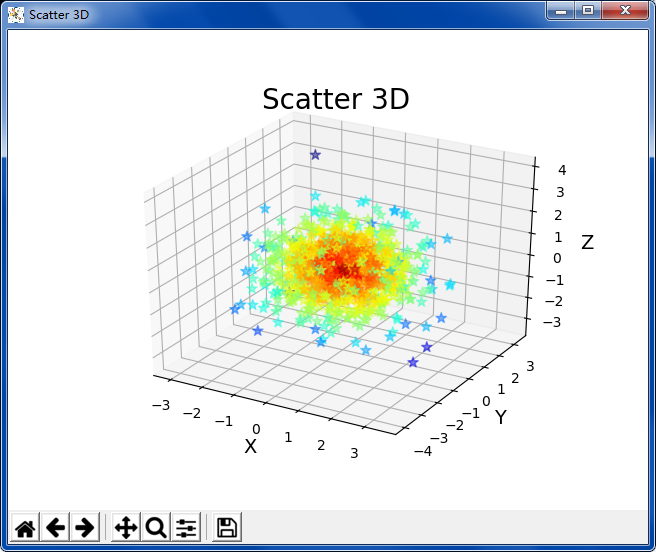### 二、极坐标系

1、格式
ax = mp.gca(projection=‘polar’)
mp.plot…
mp.scatter…
x、y分别对应极角、极径

2、测试代码

import numpy as np
import matplotlib.pylab as mp

t = np.linspace(0, 2 * np.pi, 1001)  # 极角
# 螺旋线
r_spiral = 0.8 * t  # 螺旋线，极径与极角是0.8倍的关系
# 玫瑰线
r_rose = 5 * np.sin(6 * t)

# 绘制图像
mp.figure("Polar", facecolor='lightgray')
ax = mp.gca(projection='polar')
mp.title("Polar", fontsize=20)  # 图像标题

# 坐标文本：极角，极径
ax.set_xlabel(r'$\theta$', fontsize=14)
ax.set_ylabel(r'$\rho$', fontsize=14)
mp.grid(linestyle=':')

# 螺旋线
mp.plot(t, r_spiral, c='dodgerblue', label=r'$\rho=0.8\theta$')
# 玫瑰线
mp.plot(t, r_rose, c='red', label=r'$\rho=5sin(6\theta)$')

mp.tick_params(labelsize=10)  # 刻度标签大小

mp.legend()
mp.show()


3、测试效果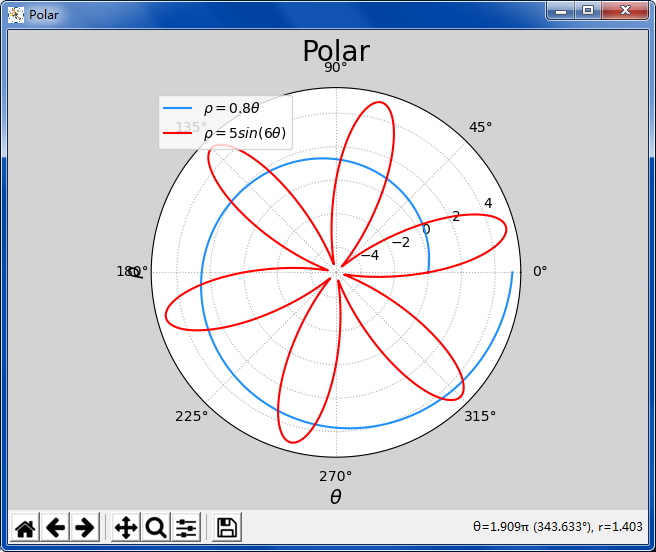### 三、半对数坐标

1、格式
mp.semilogy(…参数与mp.plot一样)
mp.semilogx()

2、测试代码

import numpy as np
import matplotlib.pylab as mp

y = np.array([1, 10, 100, 1000, 100, 10, 1])  # y坐标序列

# 绘制图像
mp.figure("Normal & Log", facecolor='lightgray')

mp.subplot(2, 1, 1)  # 创建位置在2行1列的子图

# 普通plot
mp.title("Normal", fontsize=16)  # 图像标题
mp.ylabel('Y', fontsize=12)

# 刻度定位：x方向
ax = mp.gca()
ax.xaxis.set_major_locator(
mp.MultipleLocator(1)  # 多点定位器主刻度
)
ax.xaxis.set_minor_locator(
mp.MultipleLocator(0.1)  # 多点定位器次刻度
)
# y方向
ax.yaxis.set_major_locator(
mp.MultipleLocator(250)  # 多点定位器主刻度
)
ax.yaxis.set_minor_locator(
mp.MultipleLocator(50)  # 多点定位器次刻度
)

# 设置主刻度网格线
mp.grid(which='major', axis='both', linewidth=0.75, linestyle='-', color='lightgray')
# 设置次刻度网格线
mp.grid(which='minor', axis='both', linewidth=0.25, linestyle='-', color='lightgray')
mp.plot(y, 'o-', c='dodgerblue', label='plot')
mp.legend()

mp.subplot(2, 1, 2)  # 创建位置在2行1列的子图
# 对数plot坐标
mp.title("Log", fontsize=16)  # 图像标题
mp.xlabel('X', fontsize=12)
mp.ylabel('Y', fontsize=12)

# 刻度定位：x方向
ax = mp.gca()
ax.xaxis.set_major_locator(
mp.MultipleLocator(1)  # 多点定位器主刻度
)
ax.xaxis.set_minor_locator(
mp.MultipleLocator(0.1)  # 多点定位器次刻度
)
# y方向：按照缺省来自动设置

# 设置主刻度网格线
mp.grid(which='major', axis='both', linewidth=0.75, linestyle='-', color='lightgray')
# 设置次刻度网格线
mp.grid(which='minor', axis='both', linewidth=0.25, linestyle='-', color='lightgray')
mp.semilogy(y, 'o-', c='orangered', label='semilog')
mp.legend()

mp.tight_layout()
mp.show()


3、测试效果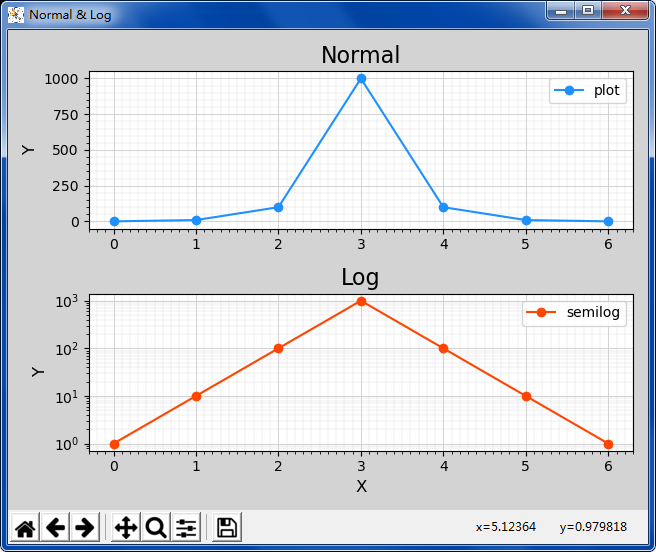展开全文python numpy 三维立体图像
• 用Matlab画三维坐标系下的点，给出x、y、z轴坐标点，用scatter3(X,Y,Z,'filled')Matlab三维点
• 三维直角坐标系与球坐标系的相互转换 球坐标为方位角，高度角和相对于半径R的高度
• ## 三维坐标系

千次阅读 2021-06-11 14:54:17
三维笛卡尔坐标系是在二维笛卡尔坐标系的基础上根据右手定则增加第三维坐标(即Z轴)...中文名三维坐标系形成基础二维笛卡尔坐标系形式种类世界坐标系、用户坐标系包含定理右手定则三维坐标系右手定则编辑在三维坐标系...

中文名

三维坐标系

形成基础

二维笛卡尔坐标系形式种类

世界坐标系、用户坐标系

包含定理

右手定则

三维坐标系右手定则

编辑

在三维坐标系中，Z轴的正轴方向是根据右手定则确定的。右手定则也决定三维空间中任一坐标轴的正旋转方向。

要标注X、Y和Z轴的正轴方向，就将右手背对着屏幕放置，拇指即指向X轴的正方向。伸出食指和中指，如图1所示，食指指向Y轴的正方向，中指所指示的方向即是Z轴的正方向。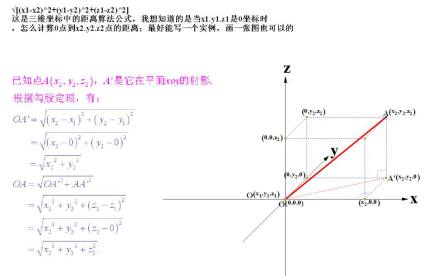图1要确定轴的正旋转方向，如图1所示，用右手的大拇指指向轴的正方向，弯曲手指。那么手指所指示的方向即是轴的正旋转方向。

三维坐标系世界坐标系

编辑

三维坐标系用户坐标系

编辑

三维坐标系三维坐标形式

编辑

1.三维笛卡尔坐标

三维笛卡尔坐标(X，Y，Z)与二维笛卡尔坐标(X，Y)相似，即在X和Y值基础上增加Z值。同样还可以使用基于当前坐标系原点的绝对坐标值或基于上个输入点的相对坐标值。

2.圆柱坐标

圆柱坐标与二维极坐标类似，但增加了从所要确定的点到XY平面的距离值。即三维点的圆柱坐标可通过该点与UCS原点连线在XY平面上的投影长度，该投影与X轴夹角、以及该点垂直于XY平面的Z值来确定。例如，坐标“10

圆柱坐标也有相对的坐标形式，如相对圆柱坐标“@ 10

3.球面坐标

球面坐标也类似与二维极坐标。在确定某点时，应分别指定该点与当前坐标系原点的距离，二者连线在XY平面上的投影与X轴的角度，以及二者连线与XY平面的角度。例如，坐标“10

同样，圆柱坐标的相对形式表明了某点与上个输入点的距离，二者连线在XY平面上的投影与X轴的角度，以及二者连线与XY平面的角度。

注：

1) 在平面直角坐标系中，分别取与x轴、y轴方向相同的两个单位向量i，j作为一组基底。a为平面直角坐标系内的任意向量，以坐标原点O为起点作向量OP=a。由平面向量基本定理知，有且只有一对实数(x，y)，使得 a=向量OP=xi+yj，因此把实数对(x，y)叫做向量a的坐标，记作a=(x，y)。这就是向量a的坐标表示。其中(x，y)就是点P的坐标。向量OP称为点P的位置向量。

2) 在立体三维坐标系中,分别取与x轴、y轴,z轴方向相同的3个单位向量i，j, k作为一组基底。若a为该坐标系内的任意向量，以坐标原点O为起点作向量OP=a。由空间基本定理知，有且只有一组实数(x，y, z)向量的坐标表示

，使得 a=向量OP=xi+yj+zk，因此把实数对(x，y, z)叫做向量a的坐标，记作a=(x，y, z)。这就是向量a的坐标表示。其中(x，y, z),也就是点P的坐标。向量OP称为点P的位置向量。

三维坐标系三维笛卡尔坐标系

三维笛卡尔坐标(X，Y，Z)是在三维笛卡尔坐标系下的点的表达式，其中，x，y，z分别是拥有共同的零点且彼此相互正交的x轴，y轴，z轴的坐标值。

三维坐标系圆柱坐标系

圆柱坐标(ρ，θ，z)是圆柱坐标系上的点的表达式。设P(x，y，z)为空间内一点，则点P也可用这样三个有次序的数ρ，θ，z来确定，其中ρ为点P在xoy平面的投影M与原点的距离，θ为有向线段PO在xoy平面的投影MO与x轴正向所夹的角。圆柱坐标系和三维笛卡尔坐标系的点的坐标的对应关系是，x=ρcosθ，y=ρsinθ，z=z。

三维坐标系球面坐标系

球面坐标系由到原点的距离、方位角、仰角三个维度构成。 球面坐标(ρ，θ，φ)是球面坐标系上的点的表达式。设P(x，y，z)为空间内一点，则点P也可用这样三个有次序的数r，φ，θ来确定，其中r为原点O与点P间的距离，θ为有向线段与z轴正向所夹的角，φ为从正z轴来看自x轴按逆时针方向转到有向线段的角，这里M为点P在xOy面上的投影。这样的三个数r，φ，θ叫做点P的球面坐标，这里r，φ，θ的变化范围为 r∈[0,+∞), φ∈[0, 2π], θ∈[0, π] . r = 常数，即以原点为心的球面； θ= 常数，即以原点为顶点、z轴为轴的圆锥面； φ= 常数，即过z轴的半平面。 其中 x=rsinθcosφ y=rsinθsinφ z=rcosθ

词条图册

更多图册

展开全文• 区域性独立坐标系三维地心坐标系之间的转换，施一民 ，陈伟，对于区域性独立坐标系三维地心坐标系之间的互相转换，提出了一种不以54国家参考椭球为过渡的转换方法，因在当前技术条件下，有�
• Android OpenGL ES (二) 绘制三维/空间坐标系 透明的立体图加三维向量和xyz坐标轴 源代码包，直接倒入eclips修改packadge便可用
• 最近很多网友表示自己不清楚几何画板如何绘制三维坐标系的操作，而本篇文章就来给大家讲解几何画板绘制三维坐标系的操作流程，大家都来一起学习吧。几何画板绘制三维坐标系的操作方法添加自定义工具。在广大版友的...

最近很多网友表示自己不清楚几何画板如何绘制三维坐标系的操作，而本篇文章就来给大家讲解几何画板绘制三维坐标系的操作流程，大家都来一起学习吧。

几何画板绘制三维坐标系的操作方法添加自定义工具。在广大版友的努力下，开发了许多可以直接使用而无须在画板中画图就可以使用的基本图形的工具，这些工具都可以加载在几何画板自定义工具下，在做课件的时候可以调用。

按住左侧工具箱“自定义工具”按钮不放，在弹出的快捷菜单中选择“3D基本工具”，在下一级菜单中有“建立三维坐标系”，这里就选择此菜单，如图所示。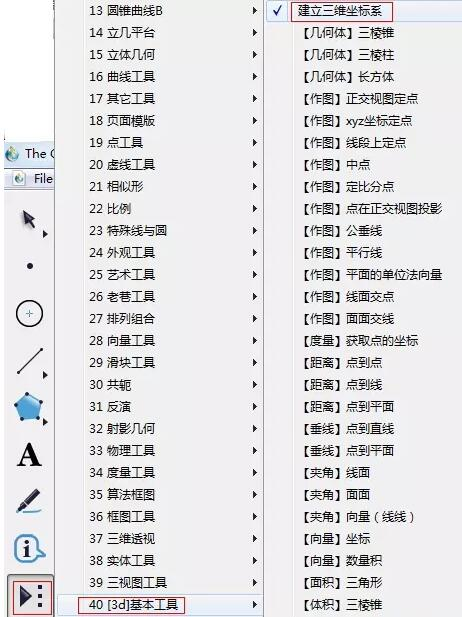选择好工具后，鼠标在画板空白区域点击，就可以画出三维坐标系，该三维坐标系工具下还包括了“三视图”、“水平和垂直旋转”、“隐藏坐标系和栅格”等操作按钮，可以演示该坐标系的三视图，且旋转该坐标系，便于观看在该坐标系下绘制的立体图形。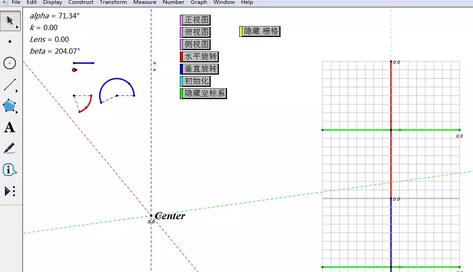若要做其他的事情，例如：绘制点，可以使用“3D基本工具”中的“正交视图定点”，该工具下包括了在三维坐标系下的基本作图，大家可以自己去慢慢尝试。

还不了解几何画板绘制三维坐标系的相关操作的朋友们，不要错过小编带来的这篇文章哦。

展开全文• 灵感的来源 下面提到的数字，单位均为毫米 ...延伸,坐标与空间直角坐标系的相互转换推导 坐标以点到极点的长度和与轴的夹角来确定一个点位置。 在空间体系中，仍然应该存在点到极点的长度。 但是角...
• 前言：接上文，使用Python双目相机标定后，通过相机的内参数矩阵与外参数矩阵计算出以左相机为原点的三维空间坐标系的坐标，此时需要使用坐标系转换，将坐标转化为指定的坐标系下的坐标。 坐标转换我目前掌握了大概...python
• 但是在网上寻找资料的时候大多是建立在二维空间的，在三维坐标系下的拟合较乱。因此在下面列举一些我所用到的直线拟合和圆拟合。 空间圆拟合 在二维空间中对圆的拟合较为简单，由初中的几何知识我们可以知道，确定...
• ## 三维坐标系旋转

千次阅读 2021-01-30 15:58:10
在做单目三维位姿估计(即估计目标物相对相机的姿态或相机相对目标物的姿态)时会用到solvepnp函数，函数原型为：cv2.solvePnP(objectPoints, imagePoints, cameraMatrix, distCoeffs[, rvec[, tvec[, ...
• 空间中三维坐标变换一般由三种方式实现，第一种是旋转矩阵和旋转向量；第二种是欧拉角；第三种是四元数。这里先介绍旋转矩阵(旋转向量)与欧拉角实现三维空间坐标变换的方法以及两者之间的关系。 这里以常见的世界...
•欧拉角转换
• 空间中三维坐标变换一般由三种方式实现，第一种是旋转矩阵和旋转向量；第二种是欧拉角；第三种是四元数。这里先介绍旋转矩阵(旋转向量)与欧拉角实现三维空间坐标变换的方法以及两者之间的关系。  这里以常见的世界...三维坐标系转换
•matlab 开发语言
•三维旋转
• 空间坐标系以及空间两三维坐标系转换矩阵解释 因为工作的原因，需要进行不同坐标系的一个转换，在查阅了众多的网页之后，发现有用的没几个，而且众多都是抄的同一篇文章，而且都是罗列以下旋转的方式，几乎没有解释...
• 反之, set nopolar ( 4.0 版中使用 unset polar 命令)可以切换到直角坐标系. 坐标下, 独立变量 t 代表角度 theta. 使用 set yrange 命令来改变图形大小. 例如: · unset parametric · set polar · plot 3...
• [含R的最终表达式]三维空间坐标系变换-旋转矩阵 https://blog.csdn.net/fireflychh/article/details/82352710... [旋转顺序zxy]三维坐标旋转矩阵 https://blog.csdn.net/lz20120808/article/details/50809397 ...计算机视觉 坐标系
• ## MATLAB 3D极坐标绘图

千次阅读 2022-04-21 14:23:12
%主要思想是把坐标转换到直角坐标系，再利用surf()函数进行绘制 %例如圆的方程坐标表达为x=r*cos(theta),y=r*sin(theta)；在区域theta=[pi/4:pi/2/100:3*pi/4],r=[1:1/50:2],由theta和r展成101*51的矩阵对应的...matlab
• 用excel绘制曲线图时如何进行坐标轴转换点"插入"->"图表"->"XY图","子图表类型"选择"无数折线散点图",点"下一步",点""->"添加",系列1的X轴输入"=Sheet1!$B$1:$B$8",Y轴输入"=Sheet1!$A$1:$A$8"；再点"添加...
• 三角测量计算三维坐标 注：三角测量须在双目相机进行立体匹配之后进行计算，针对极线校正后图像 关于极线校正可以参考我的上篇博文：相机成像+单应性变换+相机标定+立体校正 下图为立体校正后的成像平面布局： 1....计算机视觉 opencv c++ 三维重建 算法
• 上篇文章中介绍了threejs中几个基本概念，例如场景、相机、渲染器以及组件等，并通过一个简单的案例向小伙伴展示了这些东西的用法，本文来看看threejs中的坐标体系。本...
• #include <QtWidgets/QApplication> #include <vtkRenderer.h> #include <vtkRenderWindowInteractor.h> #include <vtkRenderWindow.h> #include <...vtkCubeAxesActor2D....
• 1. matlab中的三维坐标系matlab中的三维坐标系是使用的右手坐标系；输入以下代码：>> plot3(0,0,0)>> xlabel('axis X')>> ylabel('axis Y')>> zlabel('axis Z')可以看出是个很明显的右手...
• 坐标七参数转换，不同的坐标系转换和二维、三维七参数计算
• 三维坐标系之间的转换关系：R(旋转矩阵) 、T（平移矩阵）;布尔莎模型:前提是旋转角为微小旋转角;适用于大转角的罗德里格矩阵模型;算法 机器学习 计算机视觉...